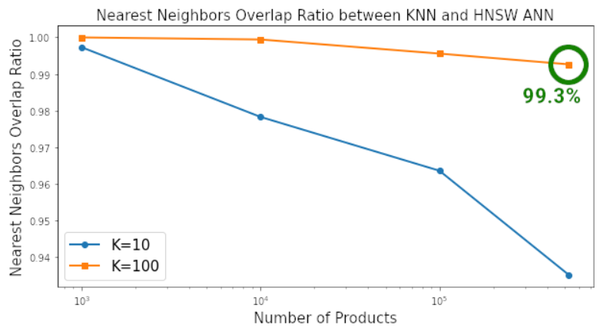# 速度數百倍之差，有人斷言KNN面臨淘汰，更快更強的ANN將取而代之 - 知乎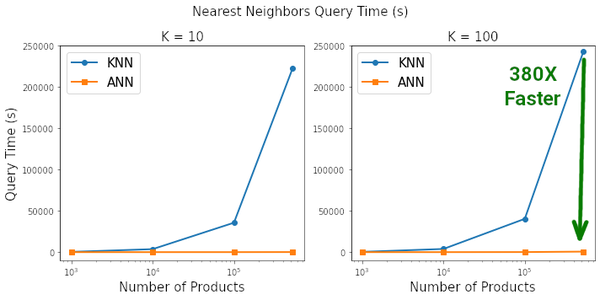KNN 簡述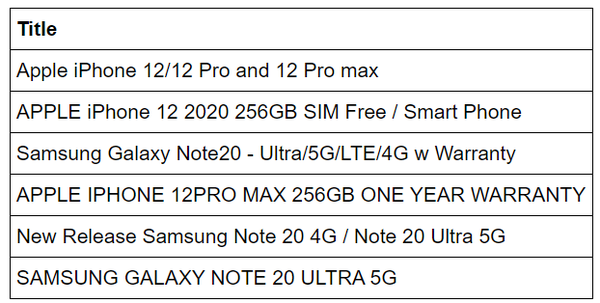KNN 存在的問題

• Spotify 的 ANNOY
• HNSW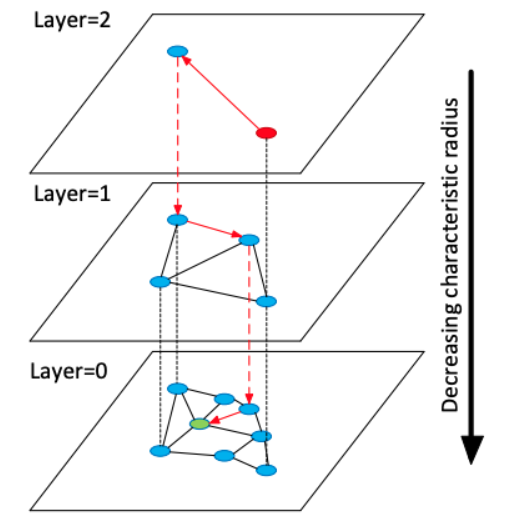HNSW 圖結構。最近鄰搜尋從最頂層開始（粗放搜尋），在最底層結束（精細搜尋）。

HNSW Python 包

``````import hnswlib
import numpy as npdef fit_hnsw_index(features, ef=100, M=16, save_index_file=False):
# Convenience function to create HNSW graph
# features : list of lists containing the embeddings
# ef, M: parameters to tune the HNSW algorithm

num_elements = len(features)
labels_index = np.arange(num_elements)    EMBEDDING_SIZE = len(features)    # Declaring index
# possible space options are l2, cosine or ip
p = hnswlib.Index(space='l2', dim=EMBEDDING_SIZE)    # Initing index - the maximum number of elements should be known
p.init_index(max_elements=num_elements, ef_construction=ef, M=M)    # Element insertion
int_labels = p.add_items(features, labels_index)    # Controlling the recall by setting ef
# ef should always be > k
p.set_ef(ef)

# If you want to save the graph to a file
if save_index_file:
p.save_index(save_index_file)

return p``````

``ann_neighbor_indices, ann_distances = p.knn_query(features, k)``

KNN 和 ANN 基準實驗

``````# Imports
# For input data pre-processing
import json
import gzip
import pandas as pd
import numpy as np
import matplotlib.pyplot as plt
import fasttext.util
ft = fasttext.load_model('cc.en.300.bin')# For KNN vs ANN benchmarking
from datetime import datetime
from tqdm import tqdm
from sklearn.neighbors import NearestNeighbors
import hnswlib``````

``````# Data: http://deepyeti.ucsd.edu/jianmo/amazon/
data = []
with gzip.open('meta_Cell_Phones_and_Accessories.json.gz') as f:
for l in f:
# Convert list into pandas dataframe
df = pd.DataFrame.from_dict(data)
df.fillna('', inplace=True)# Filter unformatted rows
df = df[~df.title.str.contains('getTime')]# Restrict to just 'Cell Phones and Accessories'
df = df[df['main_cat']=='Cell Phones & Accessories']# Reset index
df.reset_index(inplace=True, drop=True)# Only keep the title columns
df = df[['title']]# Check the df
print(df.shape)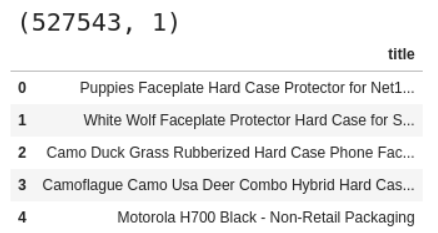亞馬遜產品資料集。

2嵌入

``````# Title Embedding using FastText Sentence Embedding
df['emb'] = df['title'].apply(ft.get_sentence_vector)# Extract out the embeddings column as a list of lists for input to our NN algos
X = [item.tolist() for item in df['emb'].values]``````

``````# Number of products for benchmark loop
n_products = [1000, 10000, 100000, len(X)]# Number of neighbors for benchmark loop
n_neighbors = [10, 100]# Dictionary to save metric results for each iteration
metrics = {'products':[], 'k':[], 'knn_time':[], 'ann_time':[], 'pct_overlap':[]}for products in tqdm(n_products):
# "products" number of products included in the search space
features = X[:products]

for k in tqdm(n_neighbors):
# "K" Nearest Neighbor search
# KNN
knn_start = datetime.now()
nbrs = NearestNeighbors(n_neighbors=k, metric='euclidean').fit(features)
knn_distances, knn_neighbor_indices = nbrs.kneighbors(X)
knn_end = datetime.now()
metrics['knn_time'].append((knn_end - knn_start).total_seconds())

# HNSW ANN
ann_start = datetime.now()
p = fit_hnsw_index(features, ef=k*10)
ann_neighbor_indices, ann_distances = p.knn_query(features, k)
ann_end = datetime.now()
metrics['ann_time'].append((ann_end - ann_start).total_seconds())

# Average Percent Overlap in Nearest Neighbors across all "products"
metrics['pct_overlap'].append(np.mean([len(np.intersect1d(knn_neighbor_indices[i], ann_neighbor_indices[i]))/k for i in range(len(features))]))

metrics['products'].append(products)
metrics['k'].append(k)

metrics_df = pd.DataFrame(metrics)
metrics_df.to_csv('metrics_df.csv', index=False)
metrics_df``````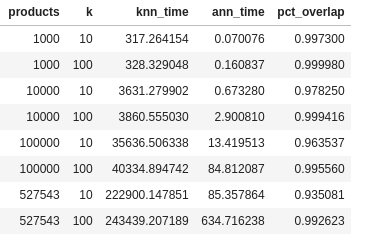以表格形式呈現的結果。